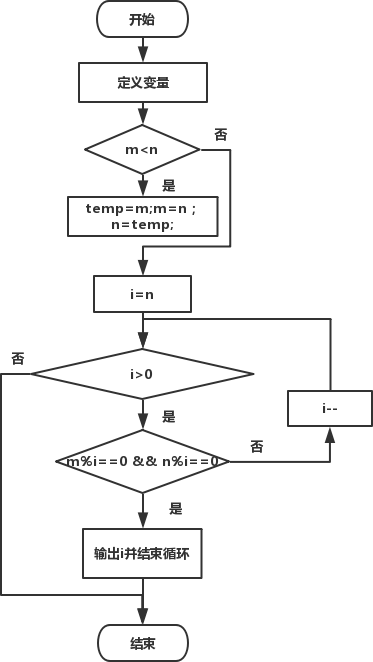• 内容
• 评论
• 相关

## 算法设计```#include<stdio.h>
int main()
{
int m, n, temp, i;
printf("Input m & n:");
scanf("%d%d", &m, &n);
if(m<n)  /*比较大小，使得m中存储大数，n中存储小数*/
{ /*交换m和n的值*/
temp=m;
m=n;
n=temp;
}
for(i=n; i>0; i--)  /*按照从大到小的顺序寻找满足条件的自然数*/
if(m%i==0 && n%i==0)
{/*输出满足条件的自然数并结束循环*/
printf("The GCD of %d and %d is: %d\n", m, n, i);
break;
}

return 0;
}```

Input m & n:100 125
The GCD of 125 and 100 is: 25

0条评论